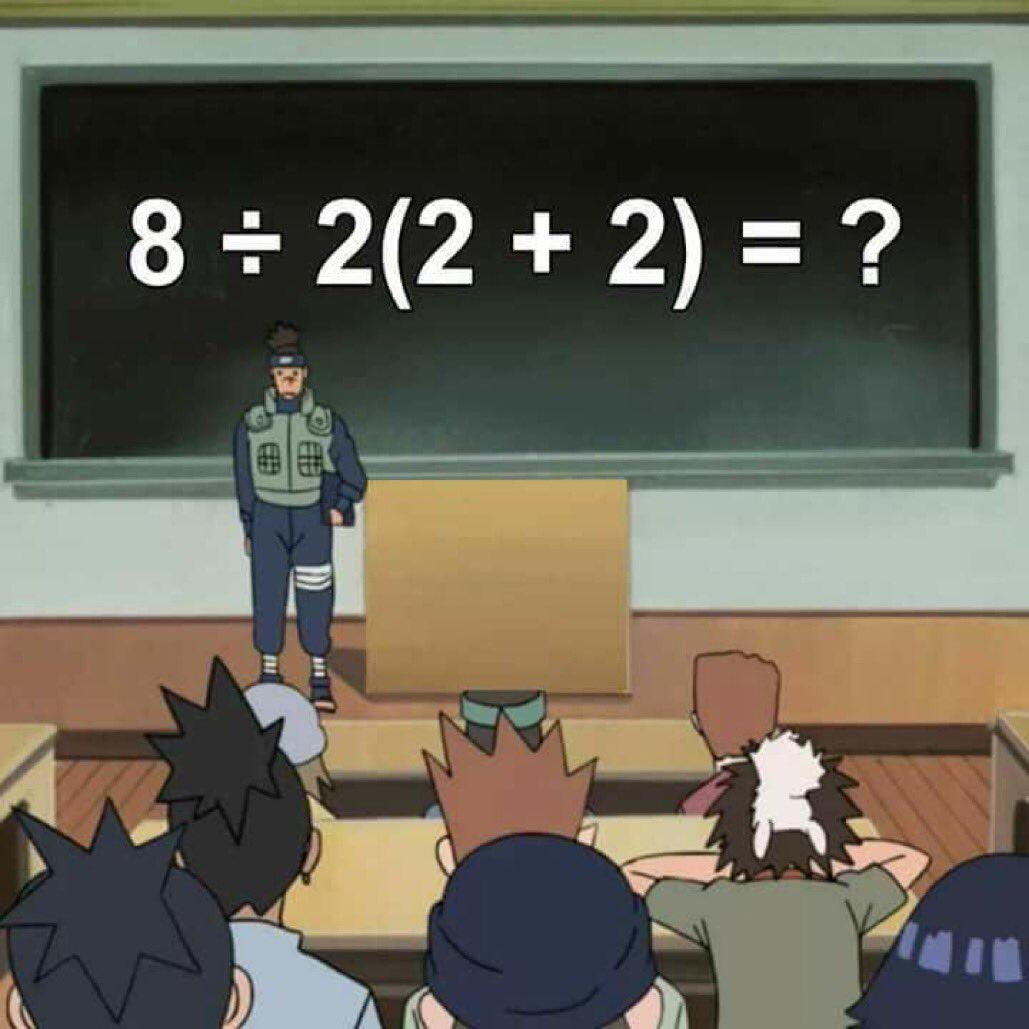# Can You Solve This Fourth Grade Math Question? Most Are Scratching Their Heads

While the years are far behind us, we’d like to think that we retained enough of what we learned that if a fourth-grader came to us for help with their homework, we’d have no problem helping them find the answer. Well if you’re confident in your abilities, you might want to rethink that. A simple fourth-grade math question has gone viral and has become a brain teaser instead.

People are divided on the answer and are passionately debating the solution. In fact, a video explaining the answer has gathered almost 20 million views! Do you think you’ve got what it takes? Let’s find out!

Are you interested in learning more about the secrets of your dreams? Tap into the 4,000-year-old science of Numerological Analysis with a FREE Numerology video report!

## No One Can Seem To Work It OutTowfiqu barbhuiya / Unsplash

Towfiqu barbhuiya / Unsplash

It turns out math is not as simple and constant as it seems. You would think that math would be one of few guaranteed things in life, as the answer of an equation would always turn out the same. Well, it looks like nothing is constant in life, so we might as well remain adaptable and in a state of flow.

People cannot get their heads around the equation. There has to be one answer though right? Let’s get our heads together and get to the bottom of it.

## Here Is The EquationOkay take a minute to try and solve this yourself first, once you have an answer, come back to compare your results. if you get it you should be pretty proud of yourself.

Those who shared their answers seem to be divided between “9” and “1.” It seems that the reason for the discrepancy in these different answers comes down to the order they complete the different steps of the equation. This is also known as the order of operations. In order to properly solve the equation and get to the correct answer, it must be calculated in the right order. If you think back to your school days, there is a standard abbreviation to help remember the order of operations known as PEMDAS.

## Let’s Get Things In Order

If you’ve forgotten what The “PEDMAS” order of operations stands for, let’s go for a quick refresher. PEDMAS stands for parentheses, exponents, multiplication, and division (left to right), and finally addition and subtraction (left to right) Some kids learn the order with the famous mnemonic “Please Excuse My Dear Aunt Sally.”

Now that we have the order mind, we should be able to solve the problem. First, we would need to tackle what’s inside the parentheses: (1+2) = 3. Okay, that’s the easy part. That leaves us with the new equation: 6÷2(3)=?

Next, since there are no exponents, let’s go to the divisions and multiplication. It should be: 6÷2 is 3, and 3(3) is 9. That should be the right answer and it is supported by Presh Talwalker in Mind Your Decisions, “This is without argument the correct answer of how to evaluate this expression according to current usage.”

## How Come So Many Think The Answer Is “1′?While we’re pretty certain the answer is 9, many continue to argue that it is actually “1.” It seems that they have confused the priority order in PEDMAS. Not only do the parenthesis come first then are followed by multiplication and division, but the equation must also be solved from left to right. Many seem to have forgotten that order.

The other problem that Talwalker highlights is that about a century ago, kids might have been taught I the ÷ symbol means that “you should divide by the entire product on the right of the symbol.” That is actually not correct in our time. Those who went by this old way would have solved the equation like this: 6÷2(3) would be 6÷(2(3)). And 6÷6=1.

## It Could Be The Way It Is Written